Dr. S. R. Lasker Library Online Catalogue

Normal view

# Statistics for business and economics / Paul Newbold, William L. Carlson, Betty M. Thorne.

Material type:TextLanguage: English Edition: 8th editionDescription: 300 pages : illustrations (some colour) ; 28 cmISBN: 9780273767060; 0132745682 (pbk); 9780132745680 (pbk); 0273767062DDC classification: 519.5 NES Online resources: WorldCat details | e-book Fulltext
Contents:
TOC Chapter 1: Describing Data: Graphical <BR>Chapter 2: Describing Data: Numerical <BR>Chapter 3: Probability <BR>Chapter 4: Discrete Random Variables and Probability Distributions <BR>Chapter 5: Continuous Random Variables and Probability Distributions <BR>Chapter 6: Sampling and Sampling Distributions <BR>Chapter 7: Estimation: Single Population <BR>Chapter 8: Estimation: Additional Topics <BR>Chapter 9: Hypothesis Testing: Single Population <BR>Chapter 10: Hypothesis Testing: Additional Topics <BR>Chapter 11: Simple Regression <BR>Chapter 12: Multiple Regression <BR>Chapter 13: Additional Topics in Regression Analysis <BR>Chapter 14: Analysis of Categorical Data <BR>Chapter 15: Analysis of Variance <BR>Chapter 16: Time-Series Analysis and Forecasting <BR>Chapter 17: Additional Topics in Sampling
Tags from this library: No tags from this library for this title.
Star ratingsAverage rating: 0.0 (0 votes)
Holdings
Item type Current library Collection Call number Copy number Status Date due Barcode Item holdsE-Book
E-book
Non-fiction 519.5 NES 2013 (Browse shelf(Opens below)) Not For LoanText
Reserve Section
Non-fiction 519.5 NES 2013 (Browse shelf(Opens below)) C-1 Not For Loan 25604Text
Circulation Section
Non-fiction 519.5 NES 2013 (Browse shelf(Opens below)) C-2 Available 26044
Total holds: 0
##### Browsing EWU Library shelves, Shelving location: E-book Close shelf browser (Hides shelf browser)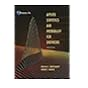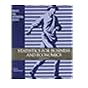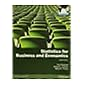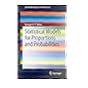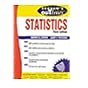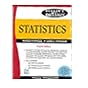519.5 MOA 2003 Applied statistics and probability for engineers / 519.5 MOI 1974 Introduction to the theory of statistics 519.5 NES 1995 Statistics for business & economics / 519.5 NES 2013 Statistics for business and economics / 519.5 SES 2013 Statistical models for proportions and probabilities / 519.5 SPS 1999 Schaum's outline of theory and problems of statistics / 519.5 SPS 2008 Schaum's outlines statistics

Includes bibliographical references and index.

TOC Chapter 1: Describing Data: Graphical <BR>Chapter 2: Describing Data: Numerical <BR>Chapter 3: Probability <BR>Chapter 4: Discrete Random Variables and Probability Distributions <BR>Chapter 5: Continuous Random Variables and Probability Distributions <BR>Chapter 6: Sampling and Sampling Distributions <BR>Chapter 7: Estimation: Single Population <BR>Chapter 8: Estimation: Additional Topics <BR>Chapter 9: Hypothesis Testing: Single Population <BR>Chapter 10: Hypothesis Testing: Additional Topics <BR>Chapter 11: Simple Regression <BR>Chapter 12: Multiple Regression <BR>Chapter 13: Additional Topics in Regression Analysis <BR>Chapter 14: Analysis of Categorical Data <BR>Chapter 15: Analysis of Variance <BR>Chapter 16: Time-Series Analysis and Forecasting <BR>Chapter 17: Additional Topics in Sampling

AS

Tahur Ahmed

There are no comments on this title.